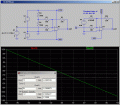# Summing amp voltage decrease. Why?

Joined Nov 13, 2015
7
I'm testing my summing amp with varying the voltage from one input and keeping my Ac signal constant and when I increase the voltage during testing the output voltage goes down. Why is that? The picture below shows my circuit and i need help as soon as possible.

Thanks.

#### bertus

Joined Apr 5, 2008
22,219
Hello,

Your summer is an inverting summer.
When the input goes higher the output goes lower.
Here is a piece of text from the page I gave you:

Much the same can be done with an inverting op-amp amplifier, using a passive averager as part of the voltage divider feedback circuit. The result is called an inverting summer circuit:Now, with the right-hand sides of the three averaging resistors connected to the virtual ground point of the op-amp’s inverting input, Millman’s Theorem no longer directly applies as it did before. The voltage at the virtual ground is now held at 0 volts by the op-amp’s negative feedback, whereas before it was free to float to the average value of V1, V2, and V3. However, with all resistor values equal to each other, the currents through each of the three resistors will be proportional to their respective input voltages. Since those three currents will add at the virtual ground node, the algebraic sum of those currents through the feedback resistor will produce a voltage at Vout equal to V1 + V2 + V3, except with reversed polarity. The reversal in polarity is what makes this circuit an inverting summer:Summer (adder) circuits are quite useful in analog computer design, just as multiplier and divider circuits would be. Again, it is the extremely high differential gain of the op-amp which allows us to build these useful circuits with a bare minimum of components.

Bertus

#### MikeML

Joined Oct 2, 2009
5,444
It is obvious that the gain is - R3/R1 = 22K/10K = -2.2. The offset (-1.038V) of the circuit you posted I determined with the sim. I suspect that this is not what you intended.

To help you understand why the offset is likely not what you want, I show the Thevinin Equivalent of your circuit. Note that V(out1) lays perfectly on top of V(out2) for the range of -5V < V(in) < 5VNotice that after calculating the Thev. Equiv., it is easy to see that the offset is -(8.48*22K/180K) = -1.04V

What gain did you want for the AC signal? Is it referenced to 0V?
What DC level did you want the AC signal riding on at the output of the opamp?

Last edited:

#### AnalogKid

Joined Aug 1, 2013
10,593
when I increase the voltage during testing the output voltage goes down. Why is that?
Because a classic summing opamp circuit is an inverter - when an input goes up, the output goes down. Your circuit has a gain of about 2, so if the AC input is 0 V, then when the DC input goes up 1 V the output is going to go down 2 V.

ak